publication

# Recursive Functions in JavaScript: 10 Examples### Miquel Canal

Tuesday 24, November 2020
• Software Engineering
• JavaScript

## What is a recursive function?

A recursive function is a function designed to call itself directly or indirectly. Using recursion, a problem can be solved by returning the value call of the same particular function.

A recursive function needs to be terminated at some point. The return of a recursive function is usually based on internal conditions which forwards the logic down to a new iteration until the base case is met.

## Base cases in recursive functions.

In recursive functions, the base case is that iteration of a recursive function that does not need an additional recursion to solve a problem.

Base cases are mandatory on recursive functions. Without a base case a recursive function will never terminate leading it to an infinite loop.

## 1 - Linear sum

Sum all values from 0 to given number and display the result.

``````function sum(number) {
if (number===0) {
return 0;
}
return number + sum(number-1);
}

sum(10);``````

## 2 - Linear sum for even numbers

Sum all even numbers from 0 to given number and display the result.

``````function sumEven(number) {
if (number===0) {
return 0;
} else if (number%2 !== 0) {
return sumEven(number-1);
}
return number + sumEven(number-1);
}

sumEven(10);``````

## 3 - Number exponent (power)

Returns the value of exponent operation from given number and grade.

``````function pow(number, power) {
if (power===1) {
return number;
}
return number * pow(number, power-1);
}

pow(2,2);``````

## 4 - Factorial of a number

Returns the product of a given number from 1 to N.

``````function factorial(number) {
if (number===1) {
return 1;
}
return number*factorial(number-1);
}

factorial(3);``````

## 5 - Pascal Triangle

Display Pascal’s triangular array of the binomial coefficients.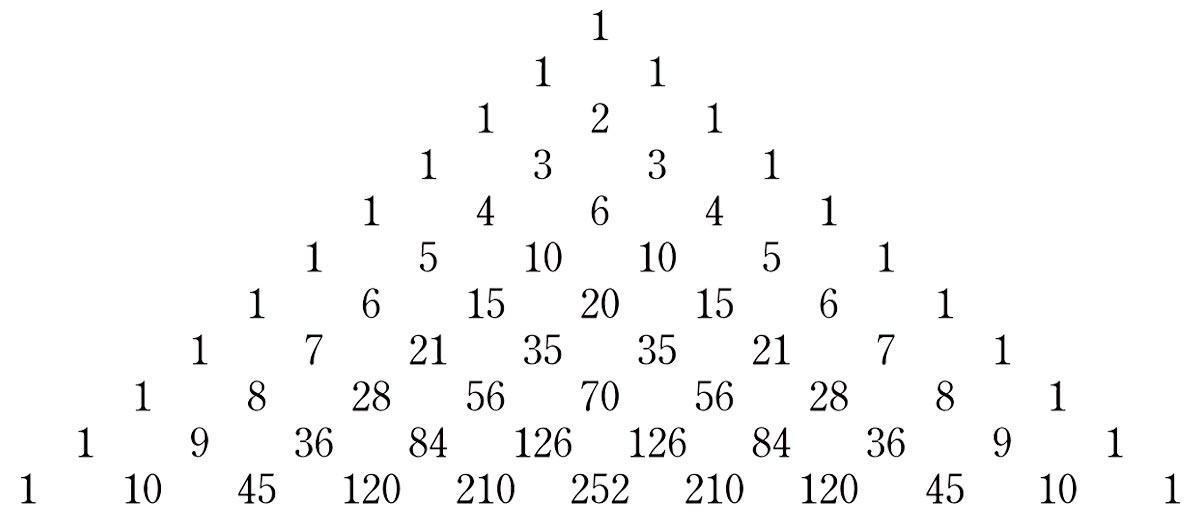``````function pascal(row, column) {
if (column>row) {
return 0;
}
if (column<=1 || row<=1) {
return 1;
}
return pascal(row-1, column) + pascal(row-1, column-1);
}

function triangle(number) {
let string = "";
for (let row=1; row<=number; row++) {
for(let column=1; column<=row; column++) {
string += `\${pascal(row, column)} `;
}
string += "\n";
}
console.log(string);
}

triangle(10);``````

## 6 - Fibonacci sequence

Displays the Fibonacci sequence from a given number starting with 0.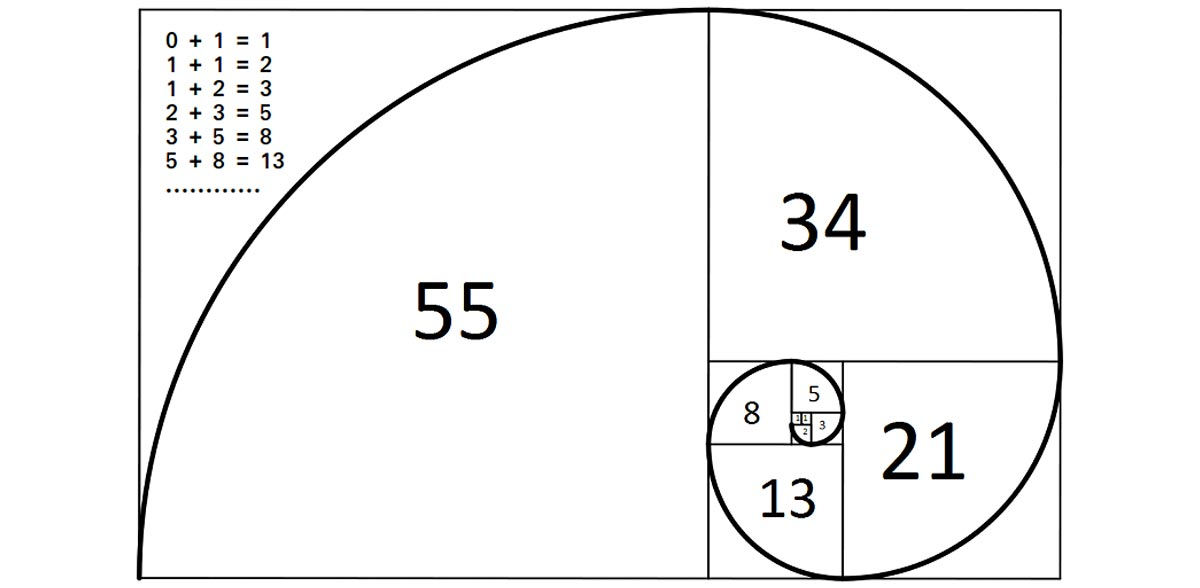``````function fib(number) {
function calc(number) {
if (number<=1) {
return number;
}
return calc(number-1) + calc(number-2);
}
let string = '';
for(let i=1; i<=number; i++) {
string += `\${calc(i)} `;
}
console.log(string);
}

fib(10)``````

## 7 - Sieve of Eratosthenes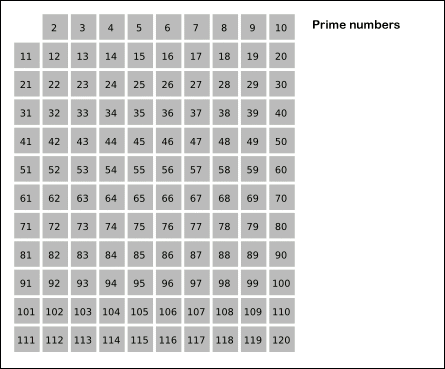An ancient algorithm for finding all prime numbers up to a given number.

``````function soe(n) {
let a = []
let p = 2
for (let i=p; i<=n; i++) {
a.push(i)
}
console.log(compose(p, a, n).filter(m => m !== 0).join('-'));
}

function compose(p, a, n) {
if (p*p > n) {
return a
}
a = a.map(m => m!==p && m%p===0 ? 0 : m)
p = a.find(m => m!==0 && m>p)
return compose(p, a, n)
}

soe(100);``````

## 8 - Object search

Search a given value on properties of nested objects.

``````function search(obj, value) {
if (typeof obj !== 'object') {
return obj === value
}

for (const key in obj) {
if (obj.hasOwnProperty(key) && search(obj[key], value)) {
return true;
}
}
return false;
}

search({
level_1: {
level_2: {
level_3: {
level_4: {
level_5: {
value: 20
}
}
}
}
}
}, 20);``````

## 9 - Leaf nodes on Binary Tree

Returns the leaf nodes of a given binary tree.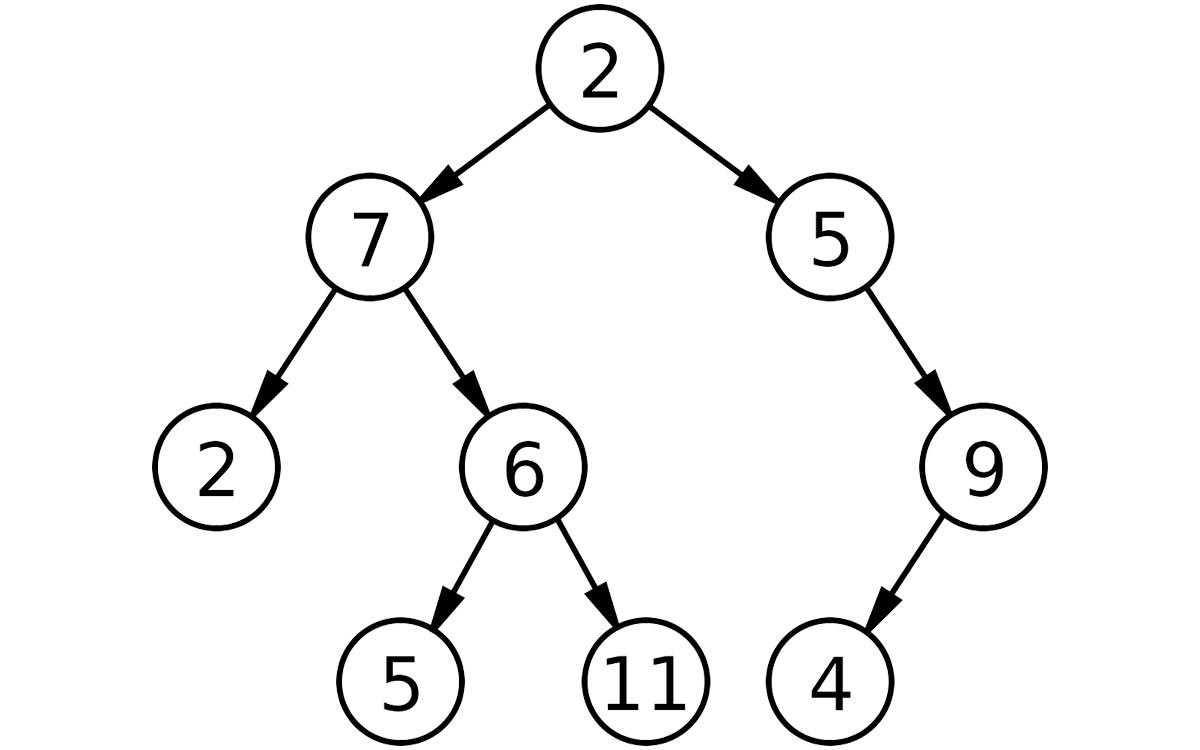``````function search_leaf(tree) {
if (!tree.left && !tree.right) {
return tree.value
}
if (tree.left) {
let l = search_leaf(tree.left)
if (l) { console.log(l) }
}
if (tree.right) {
let l = search_leaf(tree.right)
if (l) { console.log(l) }
}
}

search_leaf({
"value": 8,
"left": {
"value": 3,
"left": {
"value": 1,
"left": null,
"right": null
},
"right": {
"value": 6,
"left": {
"value": 4,
"left": null,
"right": null
},
"right": {
"value": 7,
"left": null,
"right": null
}
}
},
"right": {
"value": 10,
"left": null,
"right": {
"value": 14,
"left": {
"value": 13,
"left": null,
"right": null
},
"right": null
}
}
});
``````

## 10 - Leaf nodes on Binary Search Tree

Returns the leaf nodes on a Binary Search Tree.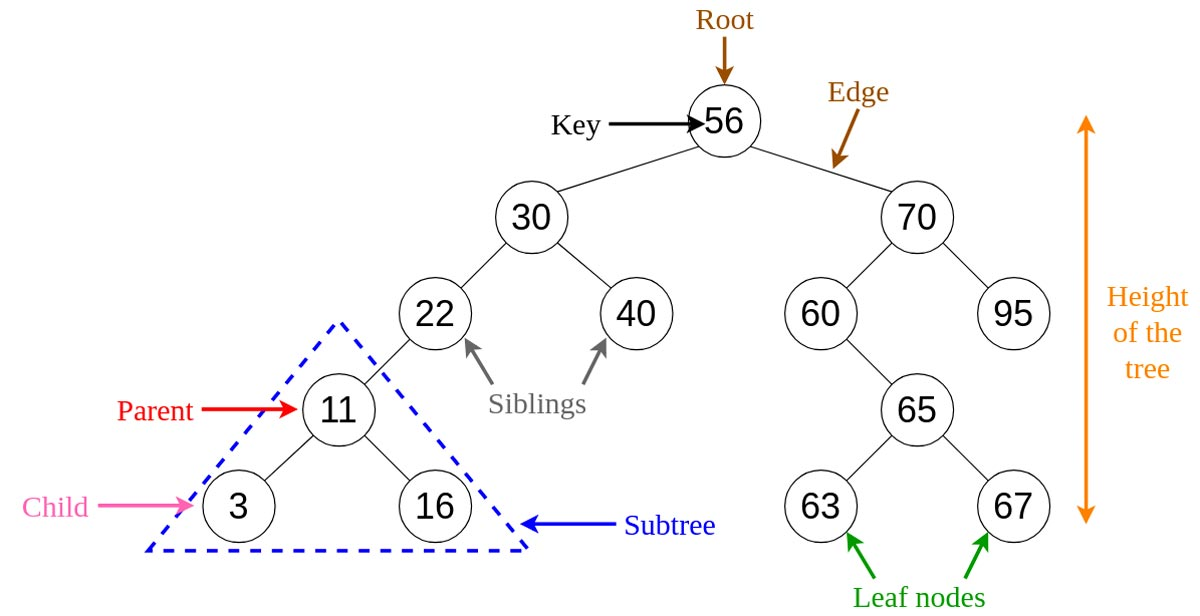``````function binary_search_tree(array) {
if (array.length===1) {
console.log(array);
return;
}
const base = array
const right = array.findIndex(n => n>base)
binary_search_tree(array.slice(1,right))
binary_search_tree(array.slice(right))
}

binary_search_tree([890,325,290,530,965]);``````

## References

This site uses cookies to ensure a great experience. By continue navigating through the site you accept the storage of these cookies.